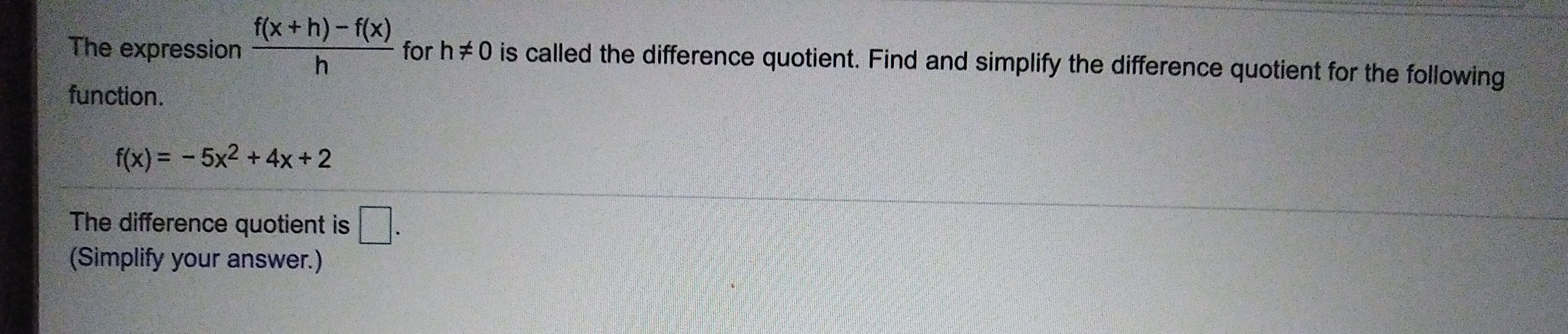f(x +h)-f(x)for hThe expression0 is called the difference quotient. Find and simplify the difference quotient for the followingfunction.5x2 + 4x + 2f(x)The difference quotient is(Simplify your answer.)

Questionhelp_outlineImage Transcriptionclosef(x +h)-f(x) for h The expression 0 is called the difference quotient. Find and simplify the difference quotient for the following function. 5x2 + 4x + 2 f(x) The difference quotient is (Simplify your answer.) fullscreen
Step 1

To evaluate the difference quotient for the giv...

Want to see the full answer?

See Solution

Want to see this answer and more?

Our solutions are written by experts, many with advanced degrees, and available 24/7

See Solution
Tagged in

Algebra